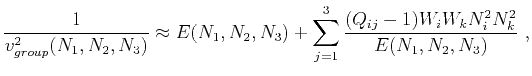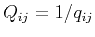On anelliptic approximations for qP velocities in TI and orthorhombic mediaNext: Proposed Approximations Up: Orthorhombic media Previous: Exact Expressions

## Extended Muir-Dellinger Approximations

Considering the Muir-Dellinger approximations for qP velocities in TI media (equations 12 and 14) and the subscript convention introduce in the first section, we can naturally extend them to orthorhombic media as follows:(41)

whereare the anelliptic parameters (in case of elliptical anisotropy),denotes velocity squared along theaxis, anddescribes the ellipsoidal part of the velocity and is defined by(42)

This extension is based on the consideration of an ellipsoid in 3D as opposed to an ellipse in 2D and the additional two anelliptic terms involvingparameters from considering a total of three symmetry planes in orthorhombic media. It is also valid to considerinstead ofbecause in consistent with the original Muir-Dellinger approximation (equation 12), only one anelliptic parameter is needed in each symmetry plane. According to the Muir-Dellinger approach, we can also derive the group-velocity approximation, which takes a similar form, with symmetric changes in the coefficients and variables as shown below:(43)

where,,,is zenith group angle (from vertical),is azimuthal group angle (from),,denotes slowness squared along theaxis, anddescribes the elliptical part of the slowness, defined by(44)

This simple extension from 2D to 3D stems from the observation that elastic wave propagation in each symmetry plane of orthorhombic media is controlled by the same Christoffel equation as in the case of TI media (Tsvankin, 2012,1997). Therefore, if anyoris zero, the extended expressions will simply reduce to the 2D Muir-Dellinger approximations for TI media in equations 12 and 14. Note that the expression in equation 41 is equivalent to the two leading terms of the phase-velocity pseudo-acoustic approximation derived by Fowler and Lapilli (2012) and Fowler et al. (2014).On anelliptic approximations for qP velocities in TI and orthorhombic mediaNext: Proposed Approximations Up: Orthorhombic media Previous: Exact Expressions

2017-04-14